### Due to the U.S. Thanksgiving holiday, please expect delayed responses during the week of 11/22.

If you have a related question, please click the "Ask a related question" button in the top right corner. The newly created question will be automatically linked to this question.

# INA216: current monitoring below 1.8V

Part Number: INA216
Other Parts Discussed in Thread: INA190, INA186

Hi there,

I'm looking for a current sensing monitor where I could monitor my load current ranging from 30uA to 10mA, the source voltage would be around 1.5V.

The INA216 would require minimum 1.8V so I guess it would not be suitable. Do you have any suitable solution to recommend?

Thanks,

HS

• Dear HS,

Unfortunately, the lowest supply available for our devices would be something like the INA190, which allows a 1.7V supply pin. This would also be the best device for sensing currents in this range, as the bias currents of the device are in the picoamp range, and therefore will mitigate error from offset.

• Hi Carolus,

Thank you for your reply. I've looked at the INA190 and have below questions:

1. I see that the INA190 has a separate Vs supply pin, so can I supply the INA190 with 3V supply while connecting my 1.5V in between my LDO and load to do the current monitoring?

2. Since the current I am monitoring is very low (from 150uA range to 10mA), so the voltage drop across the Rsense will be very small (0.01V max). Hence I could use a 1ohm sensing resistor to get a Vout ranging from 3mV ~ 2V (assuming i am using the INA190A4 with 200V/V gain) and REF connected to GND?

3. In your opinion, which would you recommend: increasing the Rsense and reduce the GAIN or reduce Rsense and increase GAIN?

4. Could INA186 also be used as a cheaper alternative to INA190?

Thank you.

Regards,

HS

• Hi HS,

1. Yes, you can do this. See section 6.3 of datasheet or figure 14.

2 and 3. The voltage drop across the shunt due to the measuring current should be some orders of magnitude higher than the input offset voltage of INA190 and the input leakage current of INA190 should be some orders of magnitude lower than the measuring current.

With a shunt of 1R 150µA gives a voltage drop of 150µA. This is only ten times higher than the input offset voltage of INA190. So for my taste Rsense is a bit too small. In any case you would need to calibrate out the offset error of INA190 in the software of your microcontroller.

On the other hand, the leakage current of 3nA is 50,000 times smaller than your measuring current, which looks ideal.

Have you thought about placing the INA190 at the input of your LDO? Here a higher voltage drop across Rsense might be more tolerable.

4. The INA186 has even a higher input offset voltage and input offset voltage drift. So the issue is even worse.

Kai

• Hi Kai,

1. With a shunt of 1R 150µA gives a voltage drop of 150µV. This is only ten times higher than the input offset voltage of INA190. So for my taste Rsense is a bit too small.

>> What would be a minimum ratio recommended ?

2. Have you thought about placing the INA190 at the input of your LDO? Here a higher voltage drop across Rsense might be more tolerable.

>> Yes, I suppose if I place the INA190 at the LDO input supplied by 3V, then I could cater for 1.2V drop across the Rsense. I could use a 200R resistor to get a max 1V drop (5mA load current), and this would be 2000 times the input offset voltage? Is this correct?

3. Going back to the INA216, is there a formula to calculate the OUT pin's voltage? Does this also means that I could use this chip and place it at the input of my LDO as point 2?

Thanks & regards,

HS

• HS,

1. You would need to define this for the purposes of your measurement. At a 10:1 ratio, this means that the offset is injecting ~10% error into your measurement. If this is good enough for the measurement you need to make, then this is fine. If you need more accuracy, then you would need to bump up the measured signal on the low end to an acceptable level. Once this is done, you need to go back and check your full scale range to make sure that your upper end is still within your linear range.

2. Kai is correct here. If you can place the measurement on the input side, you would not need to worry about the voltage consumed by the shunt in your LDO line, and the tradeoff would simply be a small reduction in the headroom of the dropout voltage.

3. The ideal output for a current sense amplifier is given by Rshunt*ILoad*GAIN, where Iload is the current under measurement flowing through the shunt. This measurement is then affected by various error sources such as offset and gain error. We have a video series on the various types of error and how to analyze these if you're interested.

Regarding the second part of your question, are you asking if you could place a chip on the input side as well as the output side to monitor both? Yes, you can do this, but as was discussed above, the shunt in the LDO output line would reduce the voltage signal from the LDO by a small amount (Rshunt*Iload)

• Hi Carolus,

Thanks for your reply. This is the first time I am using the current monitoring chip so there are a lot that I am still trying to understand here. I intend to use the INA216 / INA186 / INA190 to monitor the current drawn by my charger to determine if it is still charging or fully charged.

I've done some measurements using the INA216A4 on hand to hopefully gain a better understanding. This is used in another circuitry where the current ranges from 30uA to 60mA from a 5V supply.

1. The first two calculated Vout is above the Vsupply hence the measured Vout is kept equal to Vsupply, is that right?

2. As the load current reduces, the Calculated Vout is below the Vsupply, but there is some differences between the measured and calculated Vout, I supposed these are due to the offset / gain errors?

So if change it to my intended application, and place the current monitoring chip INA216 before the LDO, where Vsupply = 3V; Iload = 150uA ~ 5mA; Rsh=10R;

Vout = Rsh*Iload*Gain = 0.075 (min Iload) ~ 2.5V (max Iload), using the INA216A2 with 50V/V Gain. The input offset would be 20times the offset voltage; leakage current of 3uA is 50x smaller than my measured current. Do you think I should use an even bigger Rsh to get a higher ratio? But this would render my Vout at max Iload above the Vsupply?

Thanks & regards,

HS

• HS,

1. Be careful with this. You are correct in that the measurement exceeds supply, but the amplifier does not simply "output supply" in this condition. What is happening here is you are actually hitting the swing to rail limitation of the amplifier, which varies from lot to lot, and could be as low as 4.7V. You should take this headroom into account in the design, as if you exceed this condition, the amplifier will saturate and operate non-linearly. There will also be recovery time associated with this behavior when the amplifier returns to the linear region.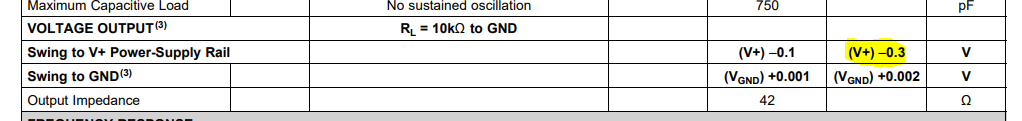2. Yes, there will be error present in the measurement. At the full scale range, the error will be dominated by the gain error (0.2% max), and at the lower end of the measurement, offset error will dominate. Other potential sources of error are noise, CMRR, PSRR, shunt resistor tolerance, temperature drifts, and more.

First, I still do not recommend the INA216 here. For the reasons I state above in point 1, I would advise against designing a setup that clearly puts you into the rail of the amplifier. With the INA190 or INA186, the input impedance of the device is sufficiently large enough that you could move down to the A3 variant, and step up the shunt into the 100's of Ωs to reap a full scale curve that meets your error budget on the low side, while still maintaining a linear measurement on the high end. Thoughts?

If we change to use the INA186 / INA190, the max Vout = Vs - 40mV = 2.96V (Vsupply = 3V) or 4.96V (Vsupply = 5V)

Based on this, I still do not see how I can increase my Rsense to a bigger value without exceeding the Vout swing limit? Below I calculated the Vout based on 10R & 100R and the Vout far exceeds the upper swing limits even with a 25 Gain? Did I miss out something here?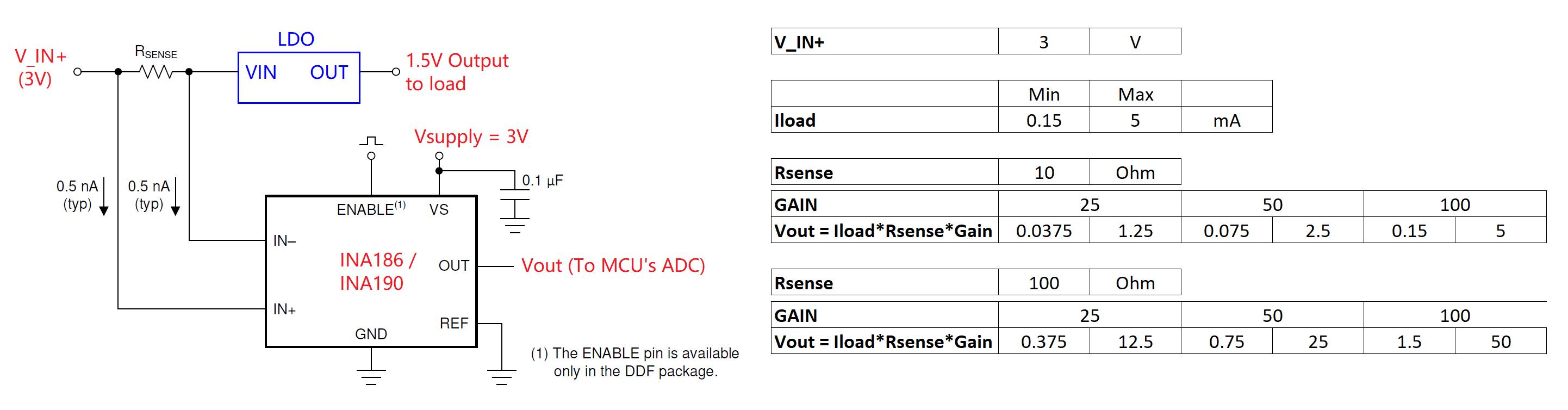Thanks & regards,

HS

• HS,

Sorry, I made this comment generally, so let me provide some additional context here. You are correct, in that the A3 is not far down enough. I think the A1 is probably the best choice here. Here's why:

First off, my recommendation of the INA186/INA190 is not for the high end of the curve, but rather the lower end. This is a pretty large current range you are looking to span here: the ratio of full scale vs. minimum input is 10mA/30uA = 333.333 . Normally, the challenge is that when trying to measure a range this wide, once you design in a shunt at the top end of the range that sufficiently plans for saturation, the signal generated by this shunt at the low end is practically noise at the low end compared to the offset and input bias currents. The INA190 exhibits bias currents in the nA/pA range, so it is the device best suited to help here on this end of the measurement.

Next, in these scenarios, its useful to keep as much sensing voltage on the front end as possible, as more sensing range equates to increased ability for the device to measure input on the front end. This means that for a wider FSR such as this, smaller gain devices are helpful. So let's jump all the way to the bottom and examine the A1 variant.

For the A1 variant of the INA190, for a Vs of 5V (4.96V swing), then the input sensing range on the front end is 4.96V/25 = .1984V. Then, for the top end of the range, to achieve this input, we would need a shunt of .1984V/.01A = 19.84Ω. Obviously this is not a standard value resistor, so we would need to either move down to the next standard value and lose a small amount of range, or move to 20Ω, and potentially risk saturation at worst case input conditions. For ease of calculation, I'll use 20Ω moving forward.

With this value, we can now look at the lower end of the curve. For this shunt, at 30uA, the generated sense voltage is 30uA*20= 600uV, and comparing this to the worst case input offset of the part, which is 15uV, then the error contributed by offset at the lower end of the curve is around 2.5%, and this would be the dominant error source at this side of the curve. PSRR would be the other large contributing factor at this level, as Vos is captured at 1.8V, and there would be several microvolts added from the supply as well. The error curve generated here shows around 5% error at the bottom end of the curve, and quickly converges as the signal grows down to <1%. Note that for the curve below I've truncated beyond 2mA as the curve has clearly converged to .27% and we want to see better granularity on the back end. :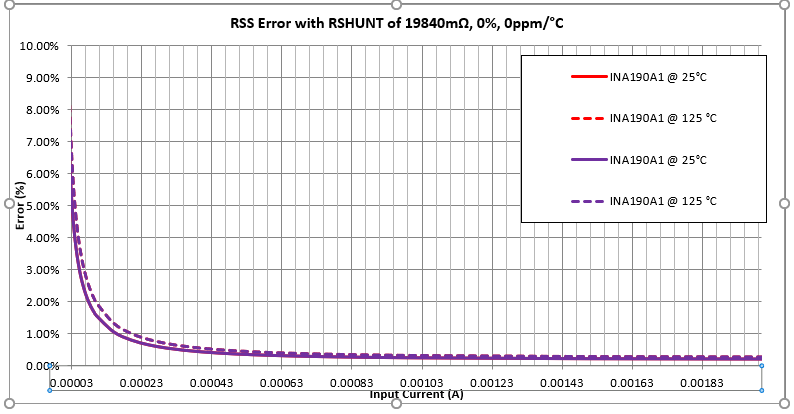Would this amount of error be sufficient?

• Hi Carolus,

Thanks for the clarifications and explanations. Based on your explanation, I update my calculations as below: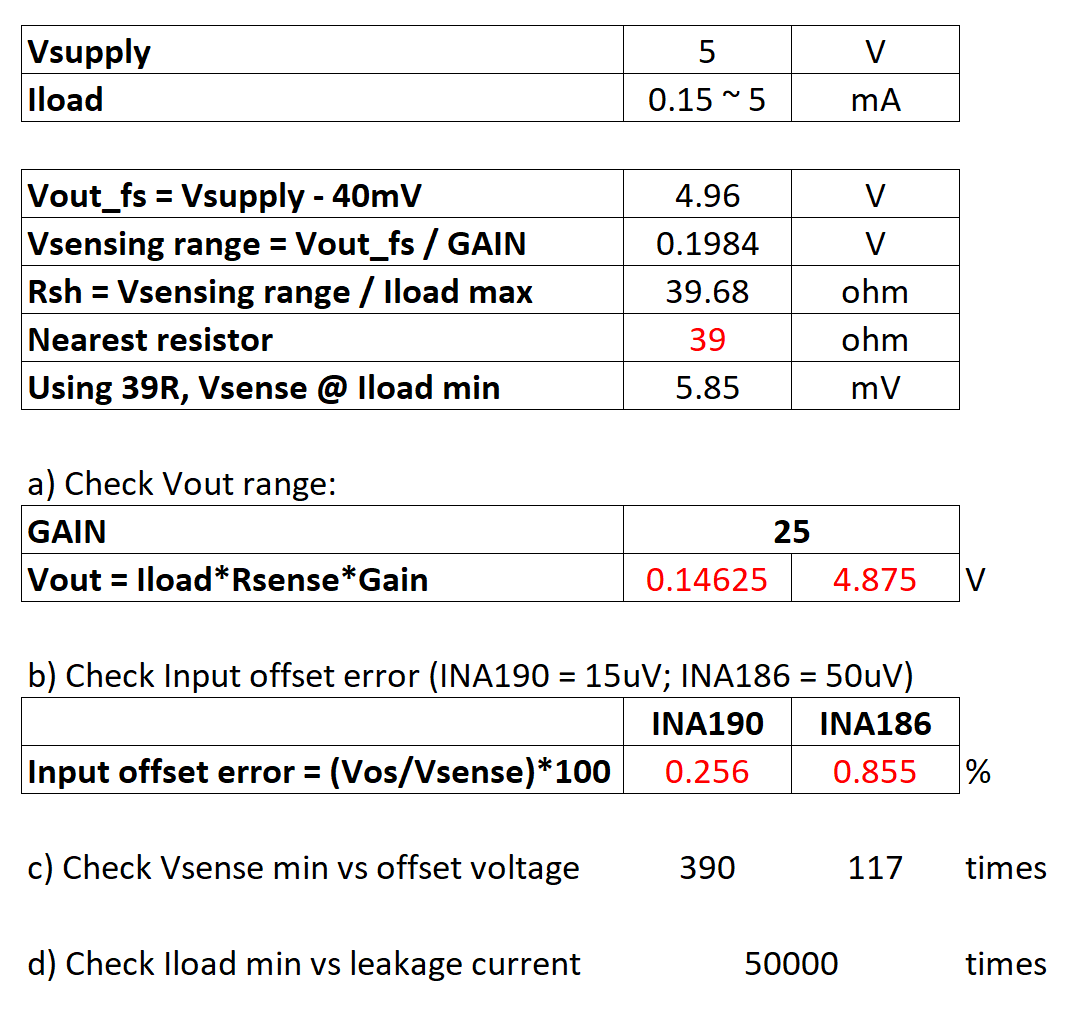So I think this should work even with INA186 considering the offset error are below 1%?

During earlier discussion, we spoke about the amplifier being saturate and enters the non-linear region when the Vout is much higher than the Vsupply. So I wonder if taking the above setting as an example, if my max Iload = 60mA, my Vout = 0.06*39*25 = 58.5V. Would this damage the INA chipset or would there be a possibility Vout outputs a voltage higher than 5V? Secton 7.4.4 in the spec mentioned about the overload but I am not sure what does it means by time-fault event?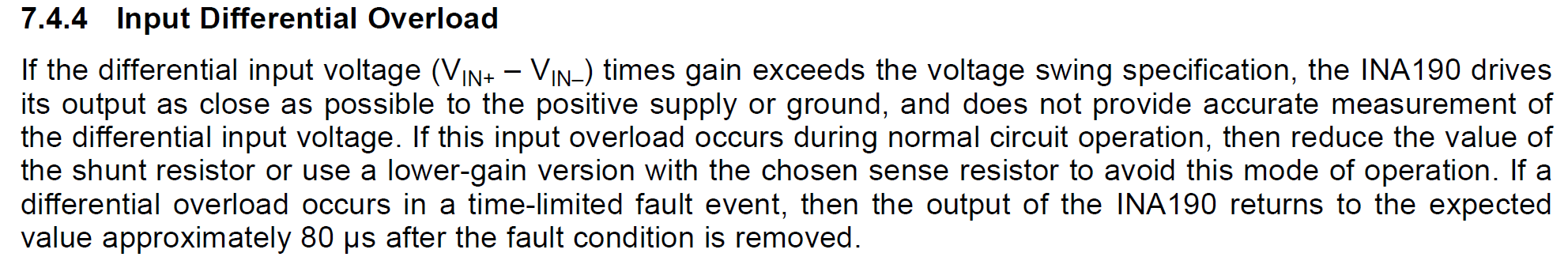Thanks & regards,

HS

• HS,

Your calculations look solid. One thing I realized this morning, this is all based on a Vs of 5V. WIll you have this voltage present in your system? We were originally discussing a Vs of 3V, which would alter the above somewhat, but the analysis would remain the same.

For the INA186 vs INA190, I believe the big question here is what is your overall error budget, i.e., what level of accuracy do you need in the measurement? Most importantly, what level of accuracy do you need in the lowest measurement, as this is where error will be the worst? The offset error for the amended range of 150uA looks reasonable, but PSRR as I mentioned above is pretty significant here. For both of these devices, Vos is quantified in the DS at a Vs of 1.8V, and the specification of PSRR for both of these devices 10uV/V. What this means is that for a Vs of 5V, there will be an additional worst case offset of 32uV present due to the supply ( (5V-1.8V)*10uV/V) that will stack with the original Vos. This is why in my original analysis the overall error at lowest value was close to 5%. This offset can be reduced by reducing Vs, but this of course reduces the sensing FSR of the system. Ultimately you need to find a healthy balance that works for your system.

Regarding your last question, the output stage is referenced to the Vs pin and GND, so no, an output greater than Vs, or less than GND is not possible. The output stages of op amps and current sense amps are usually controlled by FETs, which are always going to have some amount of collector-emitter voltage drop as an inherent consequence of the device. This is why amplifiers cannot drive directly to the rail (even "rail to rail" devices have a small amount of headroom that prevents them from truly outputting actual Vs). When a signal overdrives beyond this point, the amplifier cannot provide the voltage that is being asked of it, and the FETs enter their saturation region, hence "saturating" the amplifier. The voltage will remain at the saturated value, regardless of how much it is overdriven, but typically, this does not damage the device, only forces it into non-linearity. When the input signal returns to a value that the amplifier can provide a linear response for, it will return to linear operation, but there is usually an amount of time needed for the amplifier to snap back into the linear region (this is the time-fault event mentioned above). It's also often difficult to quantify this behavior, because it is non-linear in nature, and this can also vary part to part.

• Hi Carolus,

Yes, I've changed the Vs to 5V to have a bigger output voltage range since most likely I would have a 5V supply in the system, if this changes I would revert back to 3V and adjust the Rsh accordingly. As of now, I am still not sure what kind of accuracy I have since I have yet to lock down the ADC used. For discussion, assuming a 12bits ADC with voltage range of 2V, it would give me 0.5mV / step. So this is ~10times (INA190) or ~4.4times (INA186) above the input offset error +PSRR? Would this be good enough?

Another questions, if my application has a wide current range but I am more interested on the current detection at the lower end only, and is OK to go into saturation region when it goes above certain load current (Vout  remained at Vout fs above that load current), I could increase the GAIN or Rsh to get a better / wider Vout delta for my ADC reading? As the changes in current from max load to my min load is gradual (in minutes range), it will provide time for the amplifier to return back to its linear operation and give a better reading at low current range. Is my assumption correct or are there any other factors I need to consider?

Thanks & regards,

HS

• HS,

On the ADC side, the ADC is simply going to quantize the output of the amplifier, so I don't see the offset ratio being an issue here as you have already determined error characteristics in the design of the CSA. The total error of the quantized measurement would be the determined error in the amplifier plus whatever error comes from the ADC itself.

On your second question, this should be fine, as long as the sense voltage generated at the inputs does not exceed the absolute maximum ratings of the device, and your system can accept the delayed response in recovering to linear behavior. I would recommend testing a device in overdrive on the bench to understand the recovery behaviors and effects.

• Hi Carolus,

Thank you very much for your guidance. I will try it out using the actual part.

Regards,

HS